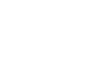How many hours,minutes,seconds in a week? - letsdiskussTrishna Dhanda

Self-Starter!!!!! | Posted | Education

# How many hours,minutes,seconds in a week?

1
0

Writer | Posted

Do you know how many seconds, minutes, and hours there are in a week? Well, there are now several calculators available online free of cost for free calculation. But it is crucial to have a good understanding of the practical calculation.

Let us first focus on the calculation of how many seconds in a week.

1/60 of a minute or 1/86400 of a day is the historical definition of a second. The duration of 9,192,631,770 periods of the radiation corresponding to the transition between the two hyperfine levels of the unperturbed ground state of the caesium 133 atoms is the SI definition of second. The abbreviation of second is sec.

There are seven days in one week. Monday, Tuesday, Wednesday, Thursday, Friday, Saturday, and Sunday are the names of the days in a week. The abbreviation of week is wk.

Both week and second are used to measure time.

So how many seconds in a week?

1Wk = 604800sec. This equation denotes that one week contains 604800 seconds.

So basically the formula is seconds = weeks x 604800.

Let this be explained with an example; suppose there are 5 weeks, so the second/5 week = 604800 × 5 = 3024000 seconds.Image Source: Google

Are you curious to know the calculation of hours in a week? Then read this carefully:

We have already given information about. We know about seven days in a week and 24 hours are there in a day.

So the ultimate question is how many hours in a week.

Hours/week = 7 x 24 = 168.

The equation concludes that there are 168 hours in one week.

Last but not the least, how many minutes in a week?

There are time converters that can help you out. Say for example, 300 min = 0.03 wk and 13,500 min = 1.3 wk, and 13500 min = 1.3wk.

1
0